# 1.材料&工具准备

• 示波器&信号源&万用表(EE三件套)
• STM32CubeIDE 1.8.0(我用的版本)
• 一些杜邦线,面包板,外围元器件等等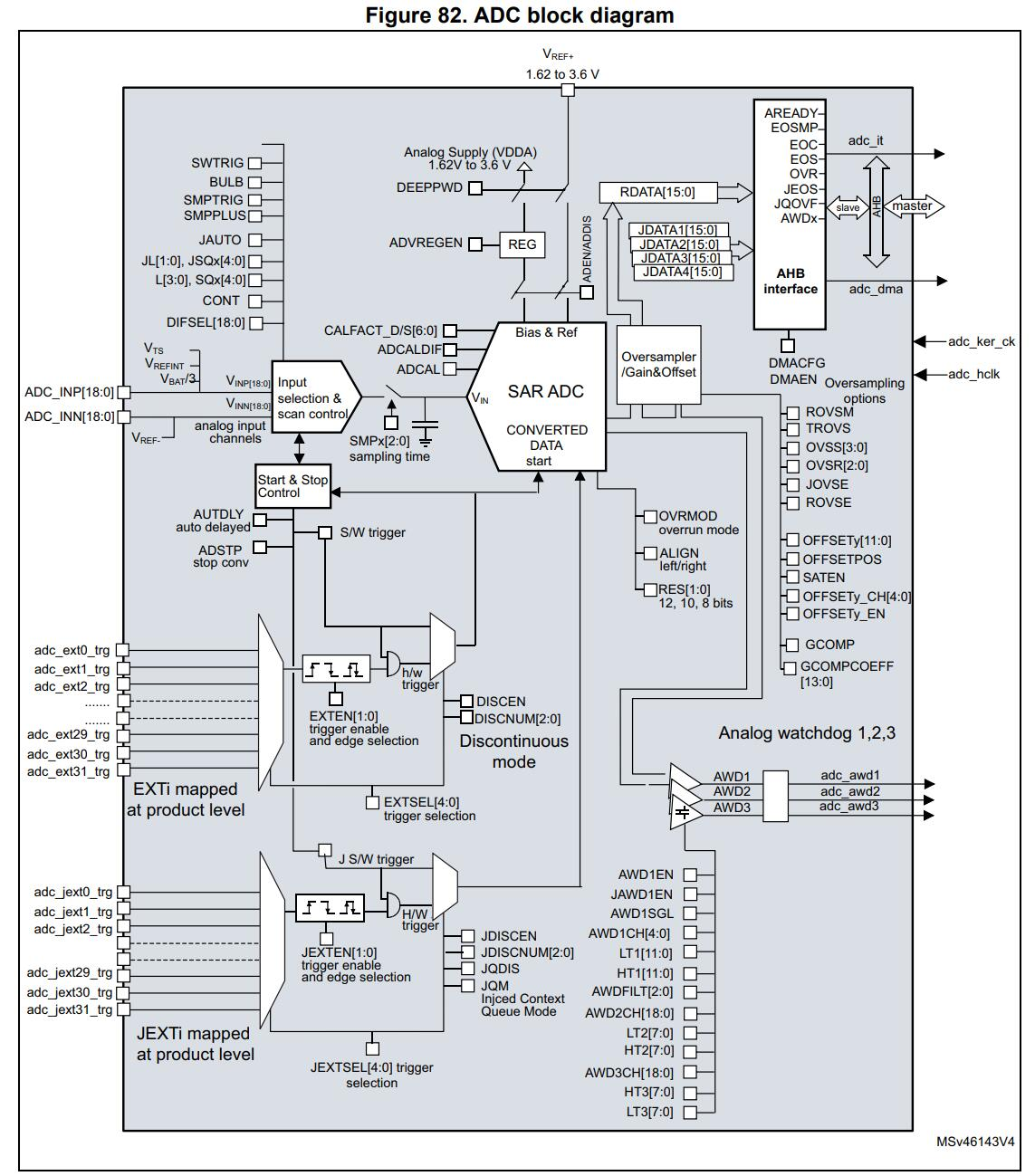• ADC时钟频率(T_{ADC_CLK})
• 采样保持电路的 采样保持时间(单位为cycles)

T_{CONV} = T_{SAMPLE}+T_{SAR} = (2.5 + 12.5) cycles = 15*25ns=375ns 

f_s = {1\over 375ns}=2.666 MHz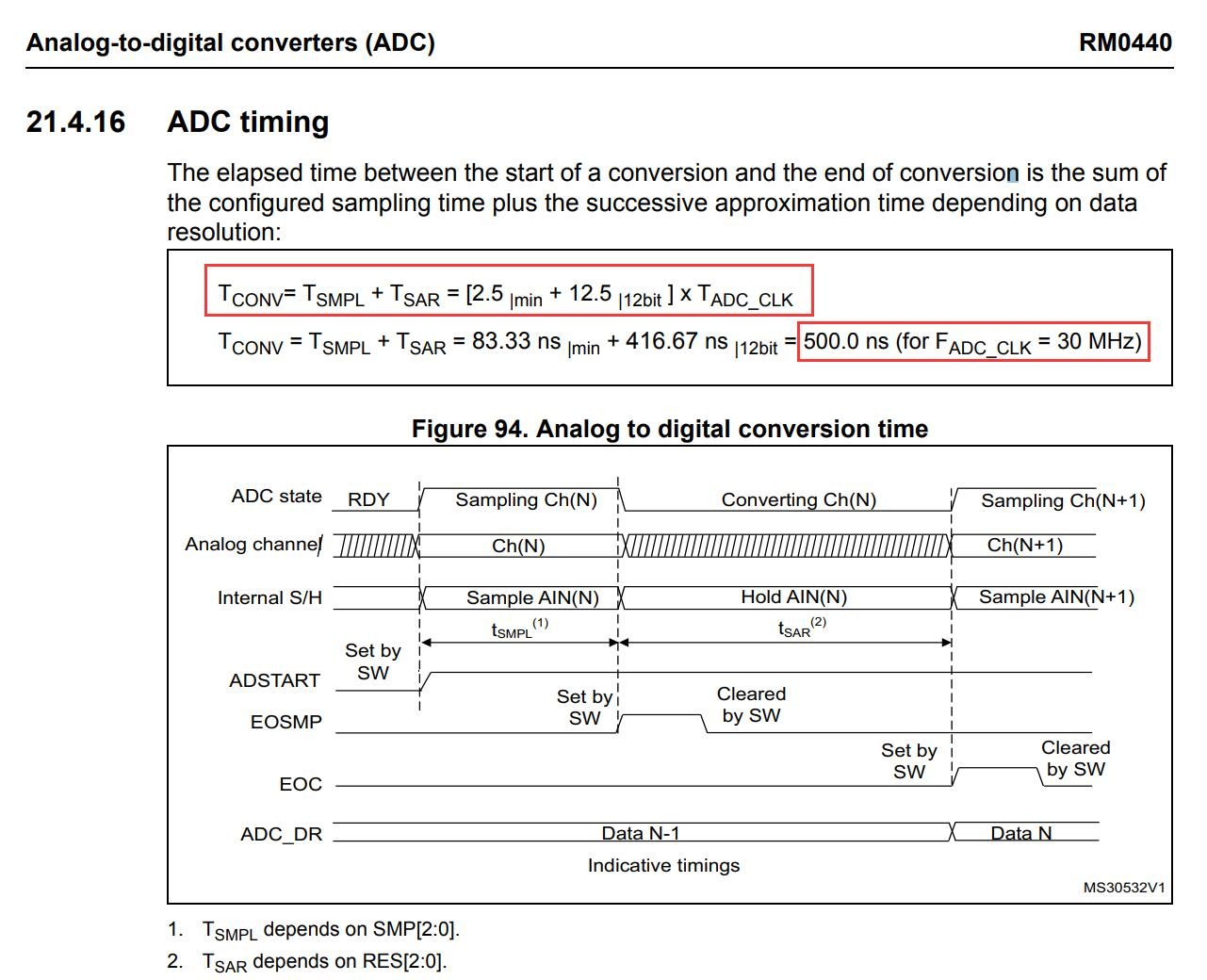## 2.3.可变采样率的实现

3. 改变采样保持时间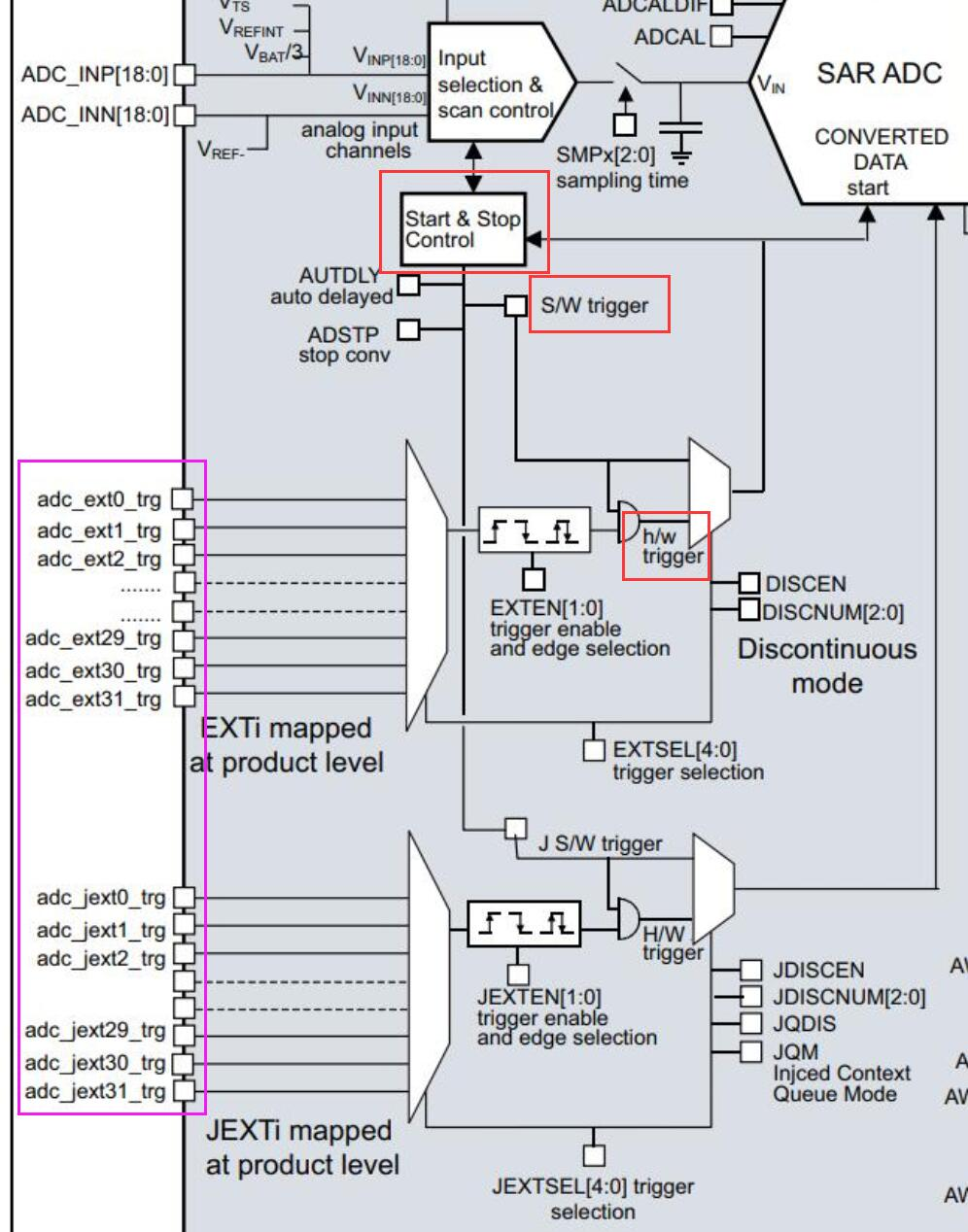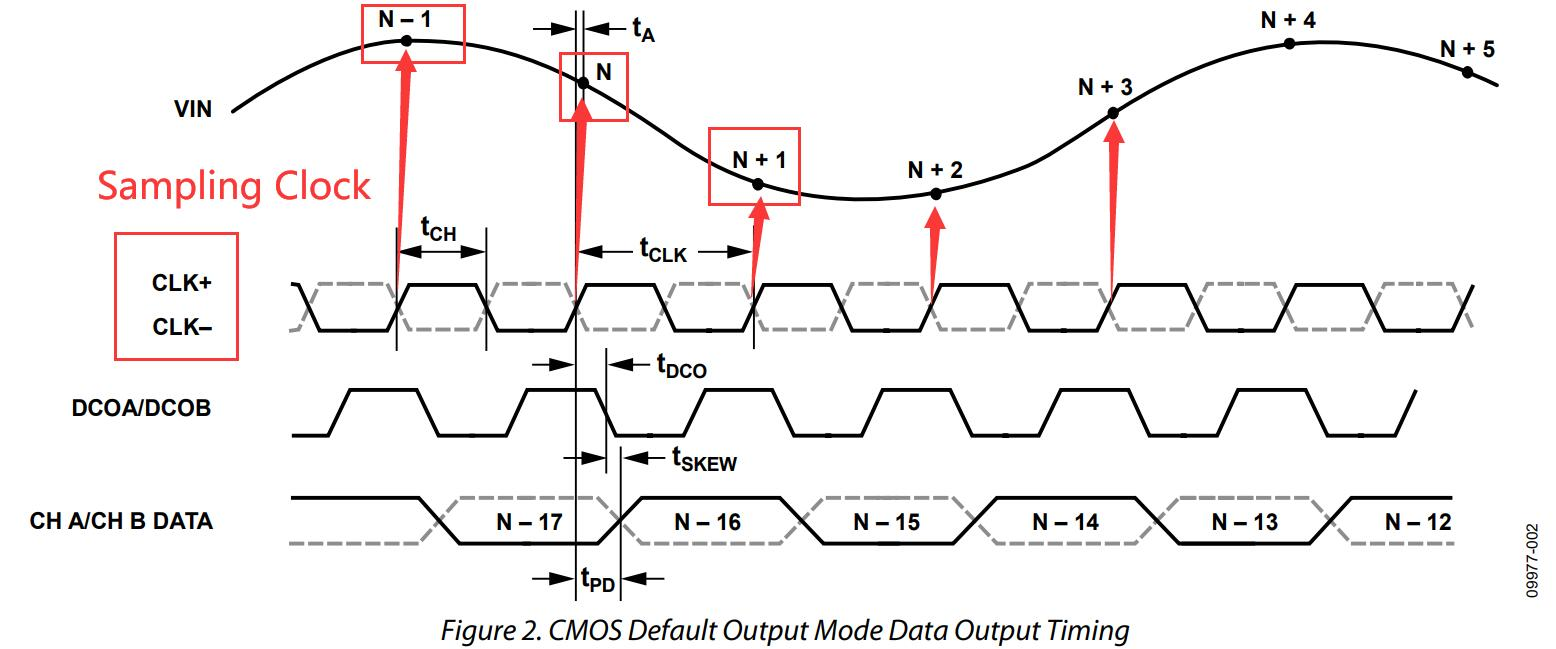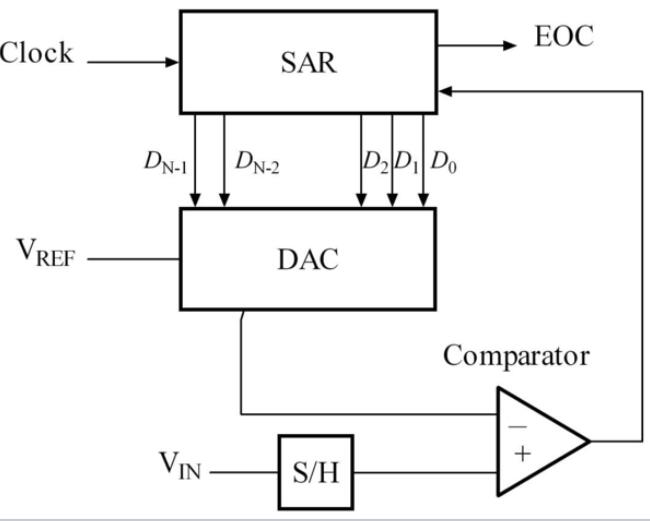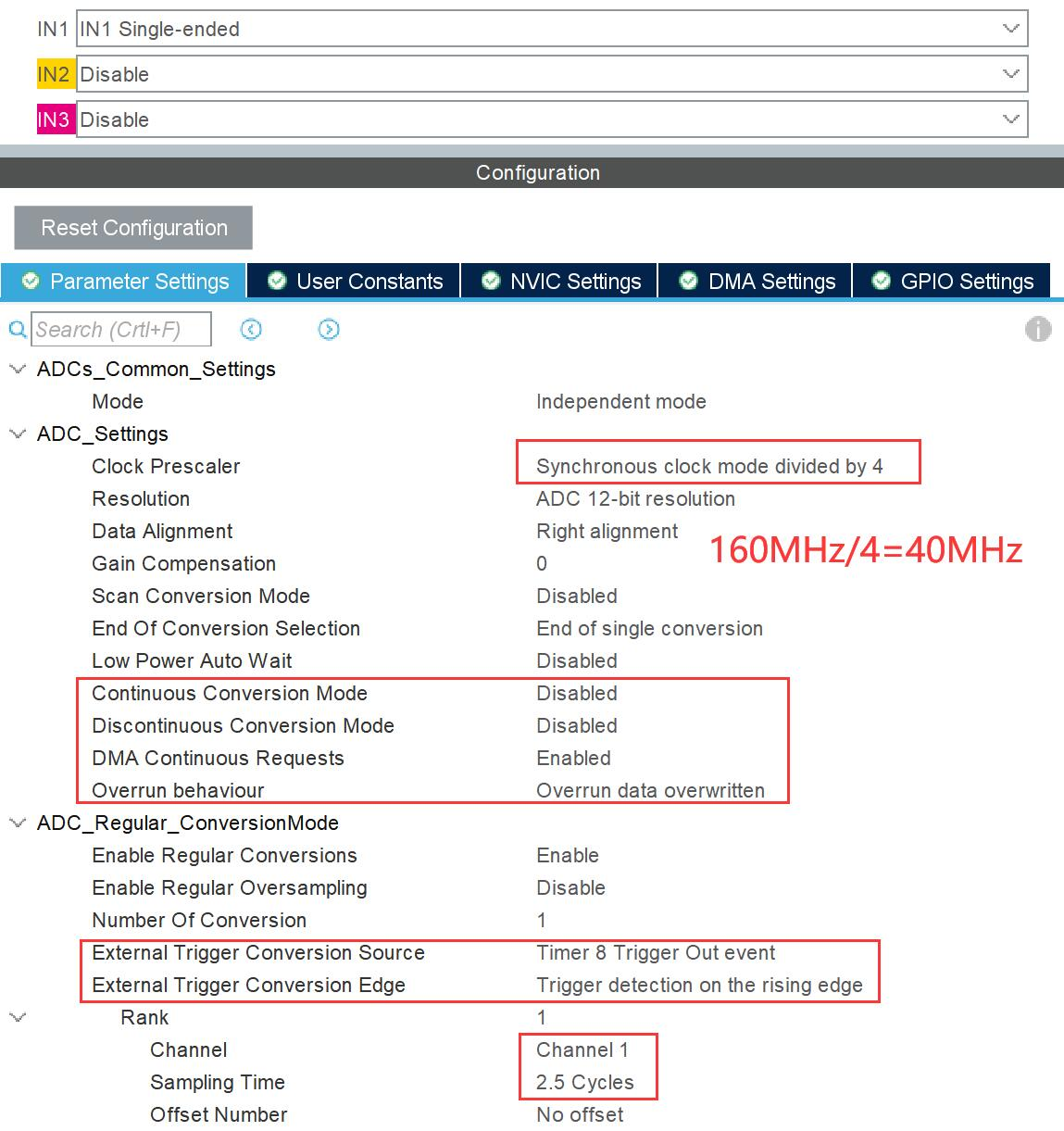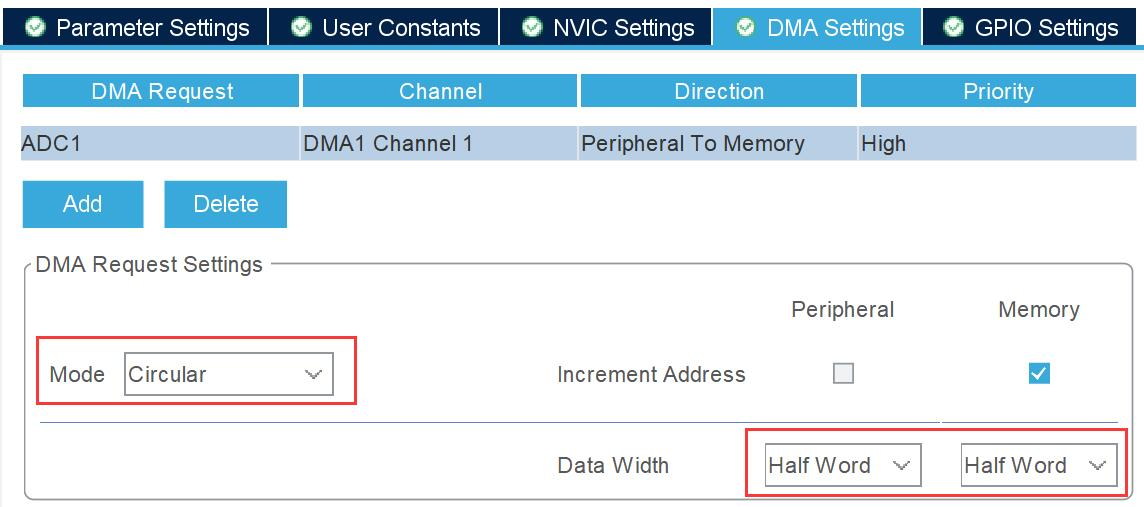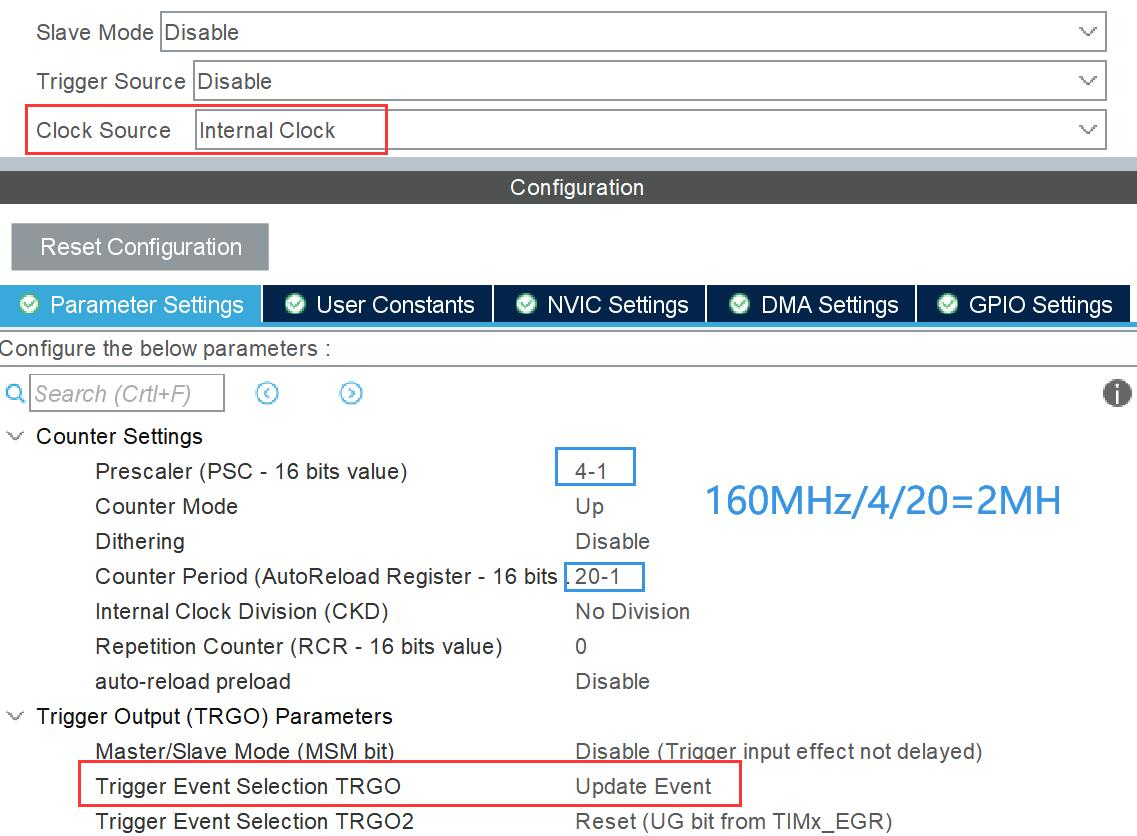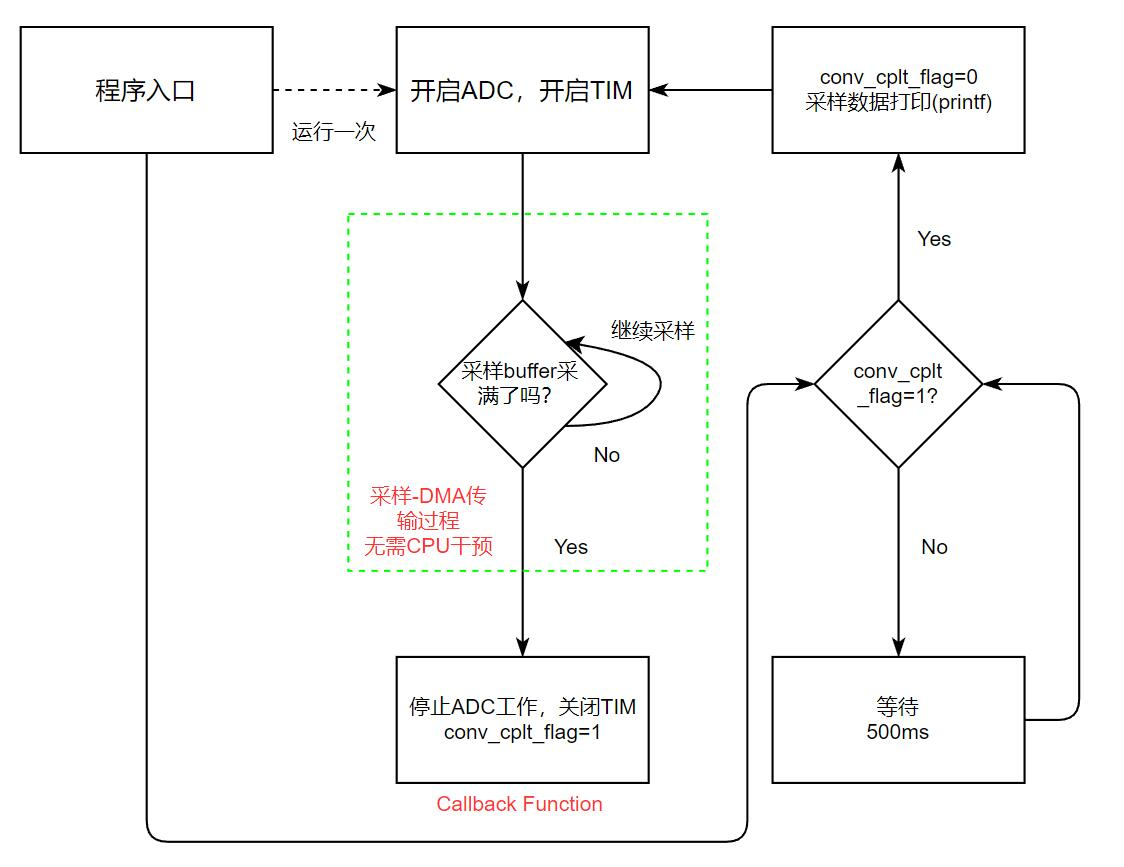/* Private user code ---------------------------------------------------------*/
/* USER CODE BEGIN 0 */
#include
//  Sample Length
//  Sample Buffer
//  Conversion complete flag

/* USER CODE END 0 */

  /* USER CODE BEGIN 2 */
//    Calibration Start & Initialize ADC
//    Delay 100ms
HAL_Delay(100);
//    Start TIM8 (Sampling Clock Source)
HAL_TIM_Base_Start(&htim8);

/* USER CODE END 2 */

/* Infinite loop */
/* USER CODE BEGIN WHILE */
while (1)
{
/* USER CODE END WHILE */

/* USER CODE BEGIN 3 */

//    If Conversion Complete
{
//    Clear cplt_flag
//    print sampled data
for(uint16_t i = 0; i < ADC_SAMPLE_LEN; i++)
{
}
//    Restart next sample cycle
HAL_TIM_Base_Start(&htim8);
}
//    "sample-print" cycle period : 500ms
HAL_Delay(500);
}
/* USER CODE END 3 */

/* USER CODE BEGIN 4 */

{
//  Stop TIM8(Sampling Clock)
HAL_TIM_Base_Stop(&htim8);
//  Set conversion complete flag
}
/* USER CODE END 4 */


/* USER CODE BEGIN 0 */
#include

#define PUTCHAR_PROTOTYPE int __io_putchar(int ch)

PUTCHAR_PROTOTYPE
{
/* Place your implementation of fputc here */
/* e.g. write a character to the USART1 and Loop until the end of transmission */
HAL_UART_Transmit(&hlpuart1, (uint8_t *)&ch, 1, 0xFFFF);

return ch;
}
/* USER CODE END 0 */

## 2.5.编译运行，检查结果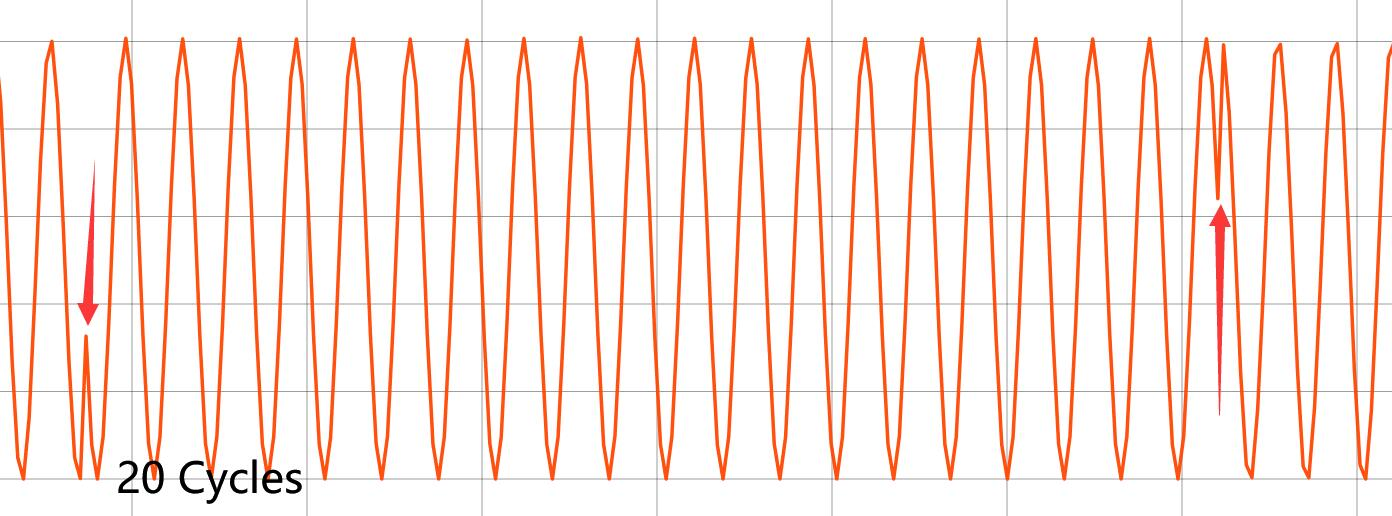STM32G474RExx具有5个SAR型ADC，并且可以通过软件配置实现各种各样的协同工作，其中就有 主-从ADC同步采样模式。配置好32的基本项，然后打开ADC1的CH1，再打开ADC2的CH2，回到ADC1的配置页面中，ADC工作模式栏发生了变化——原先只有Independent Mode Only，现在多出了2个ADC配合工作的一些模式。在这里我们选择双ADC同步采样模式(如图)，使能DMA access，并且在这里可以调整2个ADC同步采样的延迟周期，这里我们尽量希望它们没有延迟，选择最短的1 cycles，此处的cycle对应ADC的主时钟周期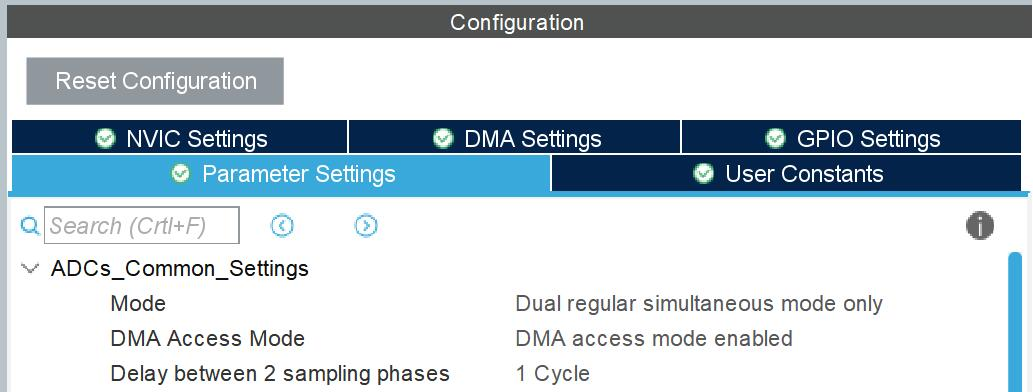/* USER CODE BEGIN PV */
#include
//  Sample Length
//  Sample Buffer
//  adc_sample for DMA access, n = SAMPLE_LEN
//  buffer for sum
//  Convert Complete flag
/* USER CODE END PV */

/* USER CODE BEGIN 2 */

//    Calibration Start & Initialize ADC
HAL_Delay(100);

//    Start TIM8 (Sampling Clock Source)
HAL_TIM_Base_Start(&htim8);

/* USER CODE END 2 */

/* Infinite loop */
/* USER CODE BEGIN WHILE */
while (1)
{
{

for (uint16_t i = 0; i < ADC_SAMPLE_LEN; i++)
{
//  Split ADC Raw Data into 2 Arrays
//  Make a sum, printf them
printf("a=%d,b=%d,c=%d\r\n",
}
//  Restart next sample cycle

HAL_TIM_Base_Start(&htim8);
}
HAL_Delay(500);
/* USER CODE END WHILE */

/* USER CODE BEGIN 3 */
}

//  Conversion Complete Callback
{
//  Stop TIM8(Sampling Clock)
HAL_TIM_Base_Stop(&htim8);
//  Set conversion complete flag
}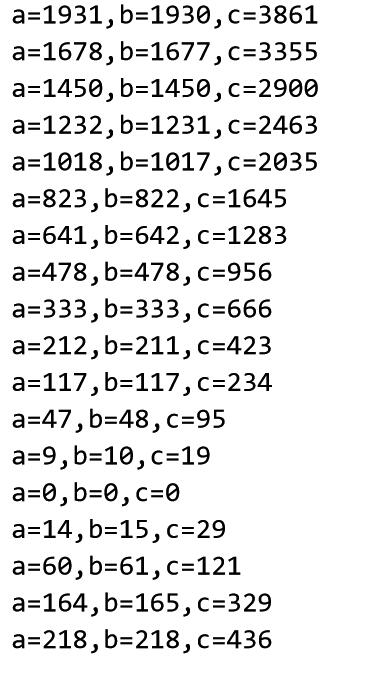# Reference

### 这篇文章有一个评论

1.第 加载中...页

看一半发现咕咕咕了？(手动滑稽